GATE  >  Architecture - AR 2014 GATE Paper (Practice Test)

# Architecture - AR 2014 GATE Paper (Practice Test) - GATE

Test Description

## 65 Questions MCQ Test GATE Past Year Papers for Practice (All Branches) - Architecture - AR 2014 GATE Paper (Practice Test)

Architecture - AR 2014 GATE Paper (Practice Test) for GATE 2023 is part of GATE Past Year Papers for Practice (All Branches) preparation. The Architecture - AR 2014 GATE Paper (Practice Test) questions and answers have been prepared according to the GATE exam syllabus.The Architecture - AR 2014 GATE Paper (Practice Test) MCQs are made for GATE 2023 Exam. Find important definitions, questions, notes, meanings, examples, exercises, MCQs and online tests for Architecture - AR 2014 GATE Paper (Practice Test) below.
Solutions of Architecture - AR 2014 GATE Paper (Practice Test) questions in English are available as part of our GATE Past Year Papers for Practice (All Branches) for GATE & Architecture - AR 2014 GATE Paper (Practice Test) solutions in Hindi for GATE Past Year Papers for Practice (All Branches) course. Download more important topics, notes, lectures and mock test series for GATE Exam by signing up for free. Attempt Architecture - AR 2014 GATE Paper (Practice Test) | 65 questions in 180 minutes | Mock test for GATE preparation | Free important questions MCQ to study GATE Past Year Papers for Practice (All Branches) for GATE Exam | Download free PDF with solutions
 1 Crore+ students have signed up on EduRev. Have you?
Architecture - AR 2014 GATE Paper (Practice Test) - Question 1

### Q. 1 – Q. 5 carry one mark each. Q. A student is required to demonstrate a high level of comprehension of the subject, especially in the social sciences. The word closest in meaning to comprehension is

Architecture - AR 2014 GATE Paper (Practice Test) - Question 2

### Choose the most appropriate word from the options given below to complete the following sentence. One of his biggest ______ was his ability to forgive.

Architecture - AR 2014 GATE Paper (Practice Test) - Question 3

### Rajan was not happy that Sajan decided to do the project on his own. On observing his unhappiness, Sajan explained to Rajan that he preferred to work independently. Which one of the statements below is logically valid and can be inferred from the above sentences?

Architecture - AR 2014 GATE Paper (Practice Test) - Question 4

If y = 5x2 + 3, then the tangent at x = 0, y = 3

*Answer can only contain numeric values
Architecture - AR 2014 GATE Paper (Practice Test) - Question 5

A foundry has a fixed daily cost of Rs 50,000 whenever it operates and a variable cost of Rs 800Q,
where Q is the daily production in tonnes. What is the cost of production in Rs per tonne for a daily
production of 100 tonnes?

Important : you should answer only the numeric value

Architecture - AR 2014 GATE Paper (Practice Test) - Question 6

Q. 6 – Q. 10 carry two marks each.

Find the odd one in the following group: ALRVX, EPVZB, ITZDF, OYEIK

Architecture - AR 2014 GATE Paper (Practice Test) - Question 7

Anuj, Bhola, Chandan, Dilip, Eswar and Faisal live on different floors in a six-storeyed building
(the ground floor is numbered 1, the floor above it 2, and so on). Anuj lives on an even-numbered
floor. Bhola does not live on an odd numbered floor. Chandan does not live on any of the floors
below Faisal’s floor. Dilip does not live on floor number 2. Eswar does not live on a floor
immediately above or immediately below Bhola. Faisal lives three floors above Dilip. Which of the
following floor-person combinations is correct?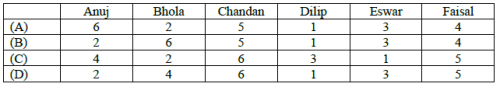*Answer can only contain numeric values
Architecture - AR 2014 GATE Paper (Practice Test) - Question 8

The smallest angle of a triangle is equal to two thirds of the smallest angle of a quadrilateral. The
ratio between the angles of the quadrilateral is 3:4:5:6. The largest angle of the triangle is twice its
smallest angle. What is the sum, in degrees, of the second largest angle of the triangle and the

Architecture - AR 2014 GATE Paper (Practice Test) - Question 9

One percent of the people of country X are taller than 6 ft. Two percent of the people of country Y
are taller than 6 ft. There are thrice as many people in country X as in country Y. Taking both
countries together, what is the percentage of people taller than 6 ft?

Architecture - AR 2014 GATE Paper (Practice Test) - Question 10

The monthly rainfall chart based on 50 years of rainfall in Agra is shown in the following figure.
Which of the following are true? (k percentile is the value such that k percent of the data fall below
that value)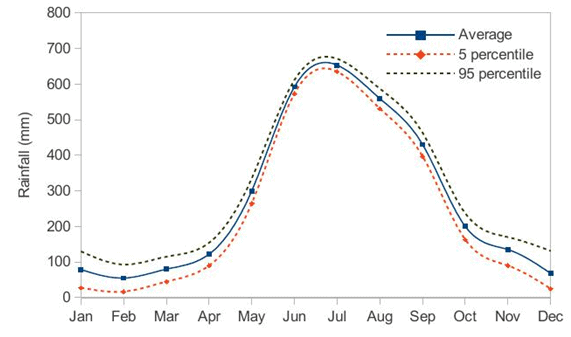(i) On average, it rains more in July than in December
(ii) Every year, the amount of rainfall in August is more than that in January
(iii) July rainfall can be estimated with better confidence than February rainfall
(iv) In August, there is at least 500 mm of rainfall

Architecture - AR 2014 GATE Paper (Practice Test) - Question 11

Q. 1 – Q. 25 carry one mark each.

Q.

Toothing is a construction technique used in

Architecture - AR 2014 GATE Paper (Practice Test) - Question 12

‘Skeleton and Skin’ concept in building design and construction evolved during the

Architecture - AR 2014 GATE Paper (Practice Test) - Question 13

As per the IRC standards, the minimum width (in m) of a two lane urban carriageway without a raised kerb is

Architecture - AR 2014 GATE Paper (Practice Test) - Question 14

Pritzker Architecture Prize 2013 has been awarded to

Architecture - AR 2014 GATE Paper (Practice Test) - Question 15

Hip roof is formed by surfaces sloping in

Architecture - AR 2014 GATE Paper (Practice Test) - Question 16

Hiroshima Peace Memorial Museum in Japan has been designed by

Architecture - AR 2014 GATE Paper (Practice Test) - Question 17

In AutoCAD, the maximum number of points which can be snapped in a circle using OSNAP command is

Architecture - AR 2014 GATE Paper (Practice Test) - Question 18

Development authorities in India are established under the provision of

Architecture - AR 2014 GATE Paper (Practice Test) - Question 19

In escalators, the angle of inclination with the horizontal plane should be in the range of

Architecture - AR 2014 GATE Paper (Practice Test) - Question 20

As per the Census of India 2011, ‘ Metropolitan Urban Agglomeration’ is a contiguous spread of several urban settlements where the minimum population size (in Lakh) is

Architecture - AR 2014 GATE Paper (Practice Test) - Question 21

BEES is an acronym for

Architecture - AR 2014 GATE Paper (Practice Test) - Question 22

In a single-stack system of plumbing

Architecture - AR 2014 GATE Paper (Practice Test) - Question 23

The maximum bending moment (kNm) in a simply supported beam of 8 m span subjected to a
uniformly distributed load of 20 kN/m (inclusive of its self-weight) over the entire span is

Architecture - AR 2014 GATE Paper (Practice Test) - Question 24

Criteria for background noise (in NC) in hospitals and apartments is

Architecture - AR 2014 GATE Paper (Practice Test) - Question 25

As per the National Building Code, the minimum width (in m) of a staircase flight in an educational
building above 24 m height should be

Architecture - AR 2014 GATE Paper (Practice Test) - Question 26

Among the following, the one that is NOT a land assembly technique is

Architecture - AR 2014 GATE Paper (Practice Test) - Question 27

The Grand Gallery in Egyptian Architecture is provided only at

Architecture - AR 2014 GATE Paper (Practice Test) - Question 28

In the Taipei 101 building, the steel sphere as TMD (Tuned Mass Damper) is suspended to reduce
horizontal sway due to

Architecture - AR 2014 GATE Paper (Practice Test) - Question 29

‘Finger Plan’ concept of urban planning was initially adopted in

Architecture - AR 2014 GATE Paper (Practice Test) - Question 30

The most important property of concrete in its fresh state is

Architecture - AR 2014 GATE Paper (Practice Test) - Question 31

An element constructed at intervals along the length of a wall to stabilize it against overturning is

Architecture - AR 2014 GATE Paper (Practice Test) - Question 32

Landscape design of Shakti Sthal, the ‘Samadhi’ of late Prime Minister Smt. Indira Gandhi, was
done by architect

Architecture - AR 2014 GATE Paper (Practice Test) - Question 33

Horizontally Wedge-shaped Treads in stairways are termed as

Architecture - AR 2014 GATE Paper (Practice Test) - Question 34

The sequence of development in a Site-and-Services scheme is

Architecture - AR 2014 GATE Paper (Practice Test) - Question 35

Which of the following is NOT a classical spatial theory of land use planning?

*Answer can only contain numeric values
Architecture - AR 2014 GATE Paper (Practice Test) - Question 36

Q. 36 – Q. 65 carry two marks each.

Q.

A housing project is proposed to be designed in a plot of 2 hectare. Maximum permissible FAR is 2. The share of the numbers of dwelling units (DU) for MIG, LIG and EWS is 1:2:3 having sizes of 55, 35 and 25 sq.m respectively. The maximum number of DU which can be accommodated in the plot is _______________

Important : you should answer only the numeric value

Architecture - AR 2014 GATE Paper (Practice Test) - Question 37

Arrange the following in ascending order of width
P. Collector Street
R. Local Street

Architecture - AR 2014 GATE Paper (Practice Test) - Question 38

Out of the following, the maximum points in the LEED (New construction) rating system can be
earned through

Architecture - AR 2014 GATE Paper (Practice Test) - Question 39

Which of the following is NOT a mechanism of bond resistance in reinforced concrete

*Answer can only contain numeric values
Architecture - AR 2014 GATE Paper (Practice Test) - Question 40

A neighborhood has 250 units of 80 sq.m each and 200 units of 100 sq.m each. If the mandatory parking requirement is one per 100 sq.m of built space then, the total area(sq.m) required for parking considering 30 percent additional area for circulation is ________________

*Answer can only contain numeric values
Architecture - AR 2014 GATE Paper (Practice Test) - Question 41

A brick wall 19 cm thick has a thermal conductivity 0.811 W/m °C. The outside and inside surface conductance of the wall are 16 W/m2 °C and 8 W/m2 °C respectively, then the U-value of the wall in W/m2 °C is ______________

Architecture - AR 2014 GATE Paper (Practice Test) - Question 42

Match the contemporary buildings in Group I with their architects in Group II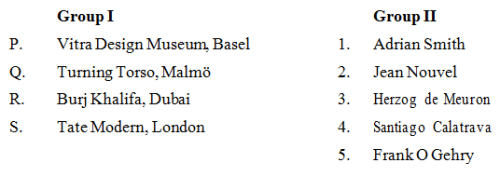Architecture - AR 2014 GATE Paper (Practice Test) - Question 43

From the following cost components of a building construction project which is not a direct cost
combination?
P. Labour cost
Q. Equipment cost
R. Material cost
S. Establishment cost
T. Supervision cost

*Answer can only contain numeric values
Architecture - AR 2014 GATE Paper (Practice Test) - Question 44

A house located in Delhi has 111 m2 of flat terrace area (runoff coefficient = 0.85) and 55 m2 of
ground area covered with grass (runoff coefficient = 0.15). If annual average rainfall is 611.8 mm,
then rain water harvesting potential (L/year) from runoff will be

Architecture - AR 2014 GATE Paper (Practice Test) - Question 45

Match the elements in Group I with the structures in Group II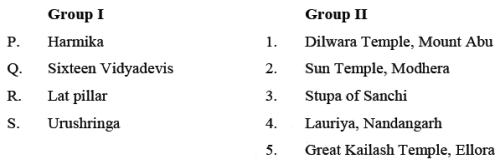*Answer can only contain numeric values
Architecture - AR 2014 GATE Paper (Practice Test) - Question 46

At a site, based on percolation test, the allowable rate of treated sewage application was determined as 65 L/m2/day. The effective depth (m) of a soak pit with a diameter of 2.5 m for the disposal of 1020 L/day of septic tank effluent is

Architecture - AR 2014 GATE Paper (Practice Test) - Question 47

Match the AutoCAD command in Group I with their functions in Group II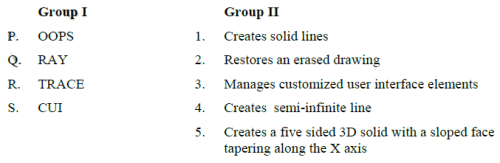Architecture - AR 2014 GATE Paper (Practice Test) - Question 48

Match the bridges in Group I with their structure type in Group II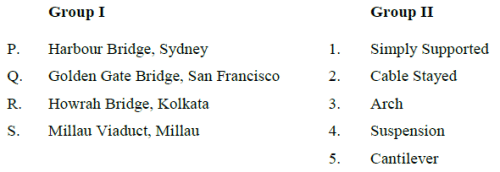Architecture - AR 2014 GATE Paper (Practice Test) - Question 49

The arithmetic average value of the sound absorption coefficient for a specific material and
particular mounting condition for four frequencies is

*Answer can only contain numeric values
Architecture - AR 2014 GATE Paper (Practice Test) - Question 50

A single span simply supported reinforced concrete beam (250 mm wide and 480 mm effective
depth) is subjected to a concentrated load of 120 kN at its mid-span. Neglecting self-weight of the
beam, the nominal shear stress (MPa) at the support section is ____________

Important : you should answer only the numeric value

*Answer can only contain numeric values
Architecture - AR 2014 GATE Paper (Practice Test) - Question 51

The optimistic time, the pessimistic time and the most likely time of a job are 6, 13 and 8 days
respectively. The variance for this job is _______________

*Answer can only contain numeric values
Architecture - AR 2014 GATE Paper (Practice Test) - Question 52

A refuse collection system consisting of two chutes is to be provided in a 20 storied residential
building with 2 flats/floor (average family size = 5) and with each chute serving one flat on each
floor. Average quantity of refuse and its density are 880 g/person/day and 240 kg/m3 respectively. If
the cleaning interval is two days, then the minimum size of the refuse container (litre) at the bottom
of each chute is _______________

Architecture - AR 2014 GATE Paper (Practice Test) - Question 53

Match the features in Group I with their architectural periods in Group II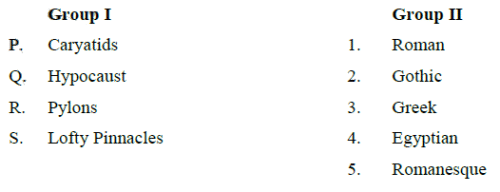Architecture - AR 2014 GATE Paper (Practice Test) - Question 54

Match the following terminologies in Group I with their descriptions in Group II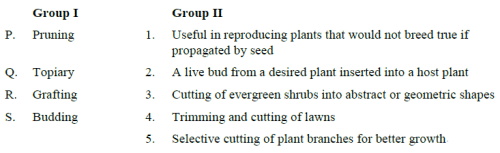*Answer can only contain numeric values
Architecture - AR 2014 GATE Paper (Practice Test) - Question 55

In a dance hall the indoor and outdoor temperatures are 28°C and 18°C respectively. There is an
internal heat gain of 5 kW and the specific heat of air (on volume basis) is 1300 J/m3 °C, then the
necessary cross sectional area (m2) of a duct with an air velocity of 2 m/s required for cooling by
ventilation is ____________

Architecture - AR 2014 GATE Paper (Practice Test) - Question 56

Assuming full compaction, strength of concrete is inversely proportional to

Architecture - AR 2014 GATE Paper (Practice Test) - Question 57

Match the terms in Group I with their examples in Group II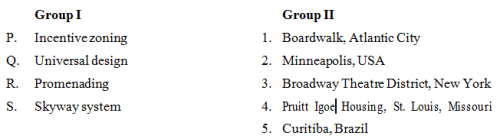*Answer can only contain numeric values
Architecture - AR 2014 GATE Paper (Practice Test) - Question 58

If yield stress of steel is 415 MPa, then strain in tensile reinforcement at the limit state of collapse
shall be at least _____________. For steel, the Young’s Modulus, E = 2 X 105 MPa.

Architecture - AR 2014 GATE Paper (Practice Test) - Question 59

Match the books in Group I with their authors in Group II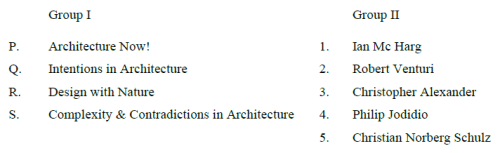Architecture - AR 2014 GATE Paper (Practice Test) - Question 60

Match the common names of the trees in Group I with their botanical names in Group II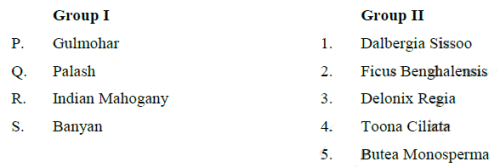*Answer can only contain numeric values
Architecture - AR 2014 GATE Paper (Practice Test) - Question 61

A room of internal dimension 4m x 5m x 3.5m (LxBxH) has 20 cm thick walls and two doors of
size 1m x 2m. The required area of Damp Proof Course (sq.m) is _____________

*Answer can only contain numeric values
Architecture - AR 2014 GATE Paper (Practice Test) - Question 62

A load of 30 kN is applied vertically downward at the free end of a cantilever of span 5 m. If the
elastic modulus of the cantilever is 30 GPa and the section has a width of 0.3 m and a depth of 0.6 m,
then, the elastic deflection (in mm) is ______________

Architecture - AR 2014 GATE Paper (Practice Test) - Question 63

Associate the plans in Group I with the options in Group II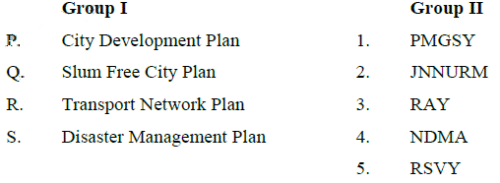*Answer can only contain numeric values
Architecture - AR 2014 GATE Paper (Practice Test) - Question 64

The capacity of a hall is 600 persons and its volume is 3000 cu.m. If an optimum reverberation time
of 1.0 second is to be achieved then the required total absorption (m2sabine) is _____________

*Answer can only contain numeric values
Architecture - AR 2014 GATE Paper (Practice Test) - Question 65

A solid straight steel rod of diameter 100 mm is bent in single curvature into a circular arc by a
moment of 50 kNm applied at its ends. If elastic modulus, E, for steel is 2 x 105 MPa, the radius of
curvature (mm) of the arc assuming π=3.14 is _____________

## GATE Past Year Papers for Practice (All Branches)

407 docs|127 tests
Information about Architecture - AR 2014 GATE Paper (Practice Test) Page
In this test you can find the Exam questions for Architecture - AR 2014 GATE Paper (Practice Test) solved & explained in the simplest way possible. Besides giving Questions and answers for Architecture - AR 2014 GATE Paper (Practice Test), EduRev gives you an ample number of Online tests for practice

## GATE Past Year Papers for Practice (All Branches)

407 docs|127 tests(Scan QR code)